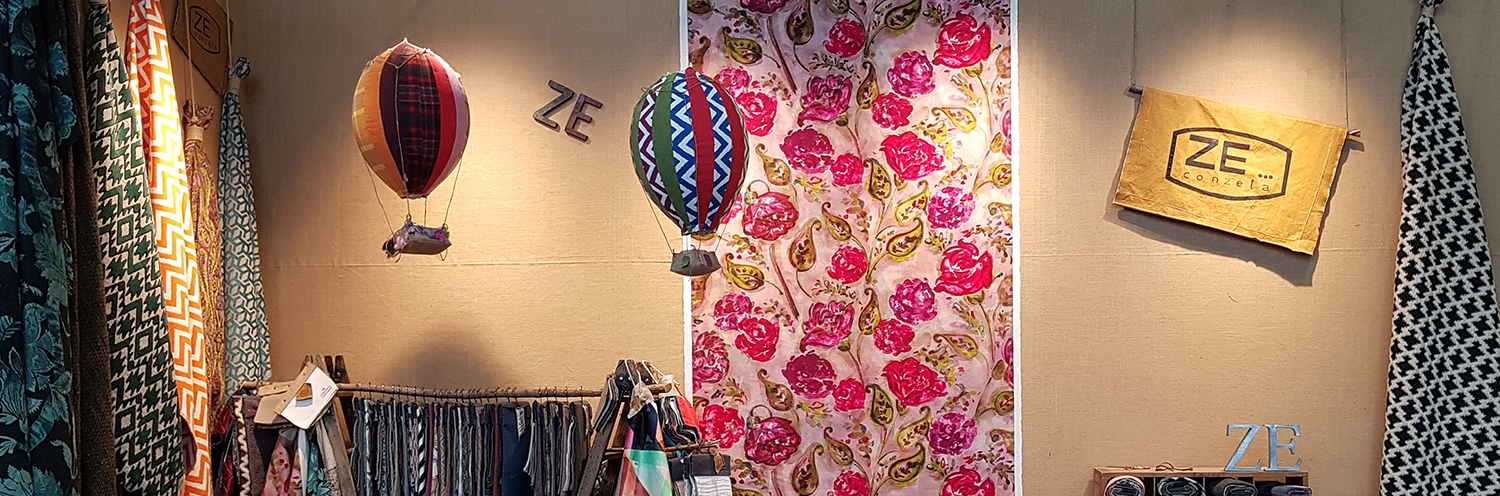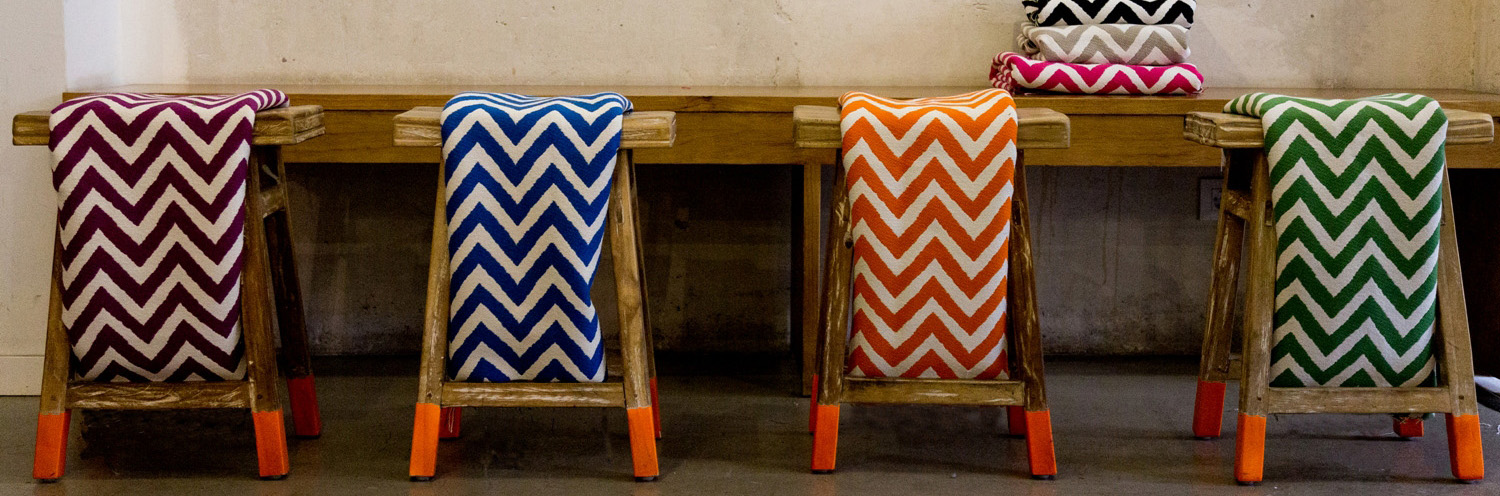# If we were a song, we would be Lennon's IMAGINE, if we were a painting, we would be THE SUNFLOWERS by Van Gogh, if we were a sea, we would be THE MEDITERRANEAN, but, because we are fabrics, we are ZE… conzeta.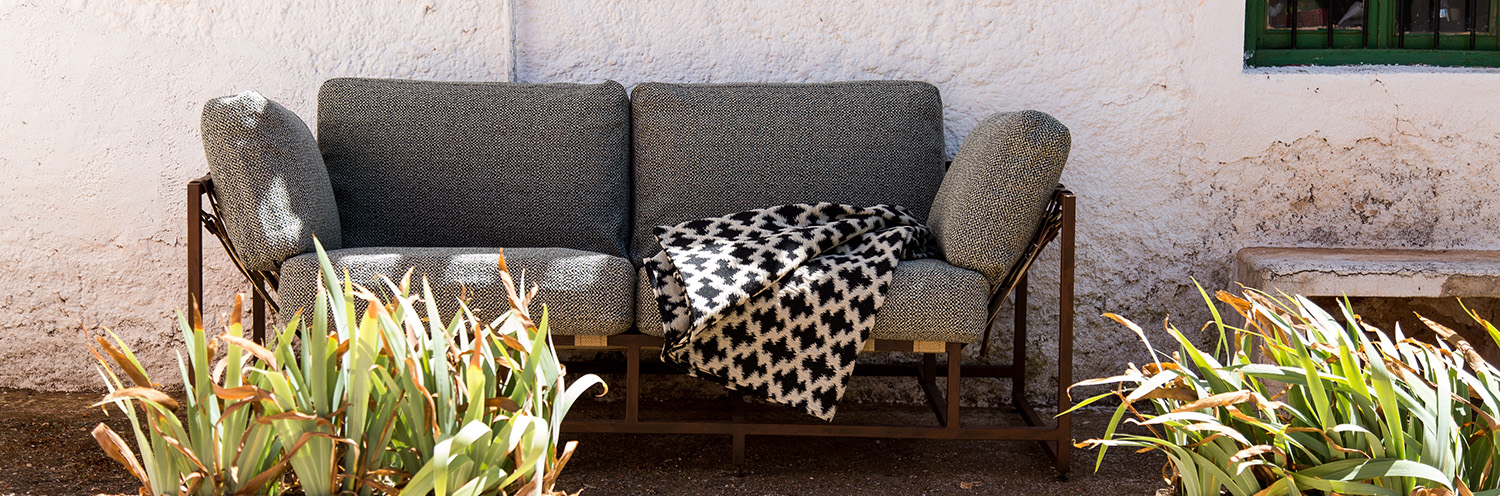# If we were a song, we would be Lennon's IMAGINE, if we were a painting, we would be THE SUNFLOWERS by Van Gogh, if we were a sea, we would be THE MEDITERRANEAN, but, because we are fabrics, we are ZE… conzeta.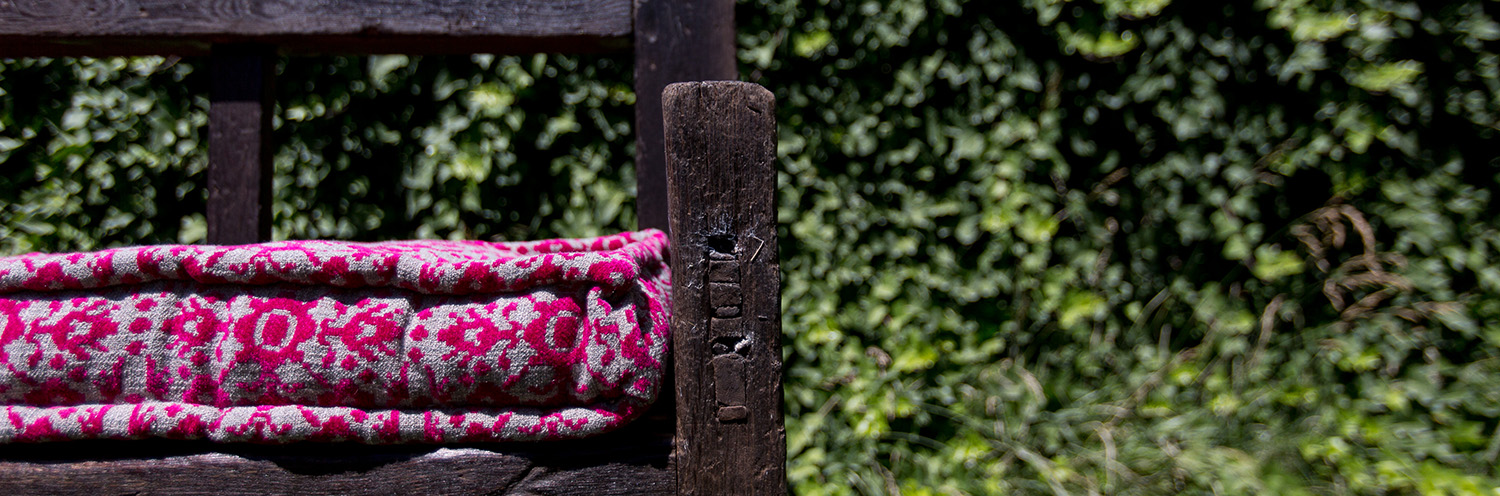# If we were a song, we would be Lennon's IMAGINE, if we were a painting, we would be THE SUNFLOWERS by Van Gogh, if we were a sea, we would be THE MEDITERRANEAN, but, because we are fabrics, we are ZE… conzeta.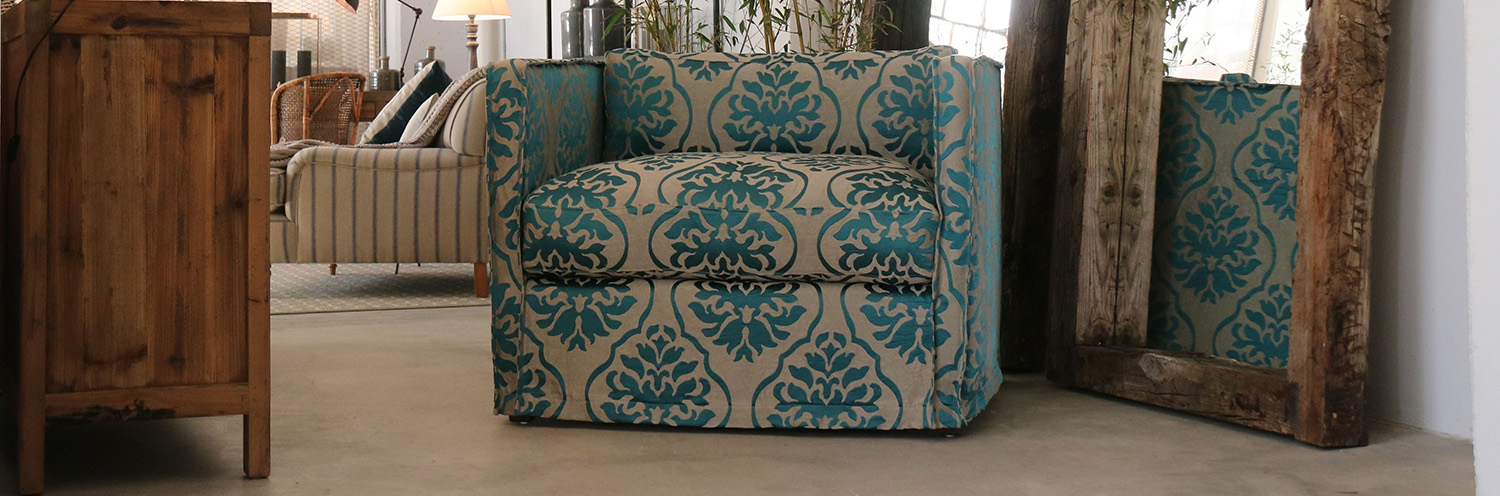# If we were a song, we would be Lennon's IMAGINE, if we were a painting, we would be THE SUNFLOWERS by Van Gogh, if we were a sea, we would be THE MEDITERRANEAN, but, because we are fabrics, we are ZE… conzeta.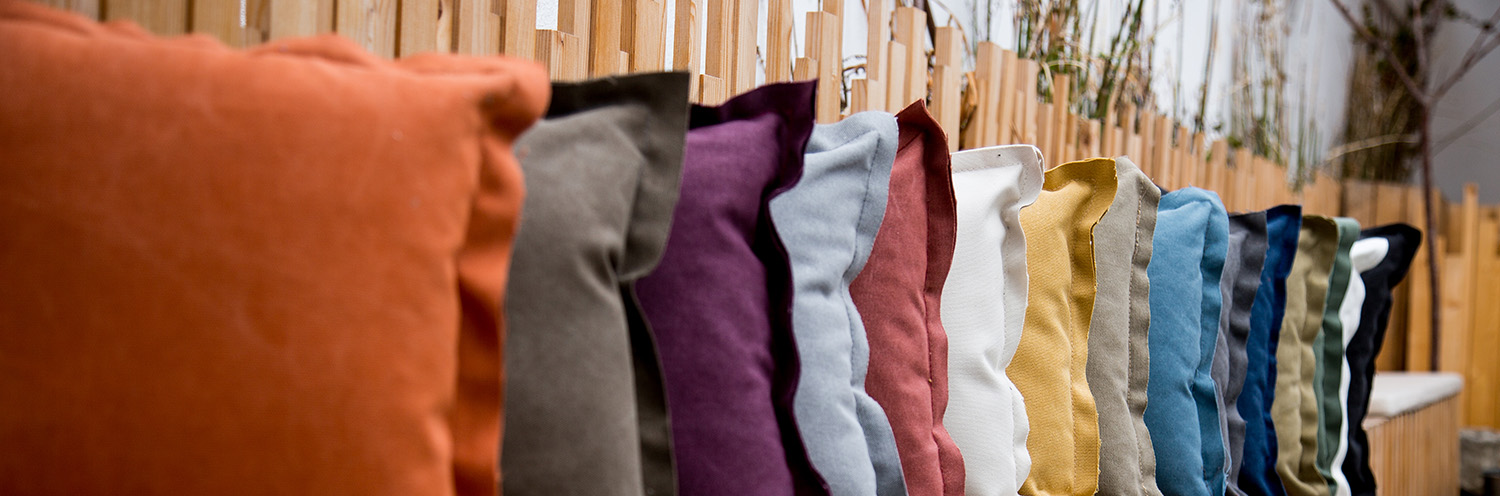# If we were a song, we would be Lennon's IMAGINE, if we were a painting, we would be THE SUNFLOWERS by Van Gogh, if we were a sea, we would be THE MEDITERRANEAN, but, because we are fabrics, we are ZE… conzeta.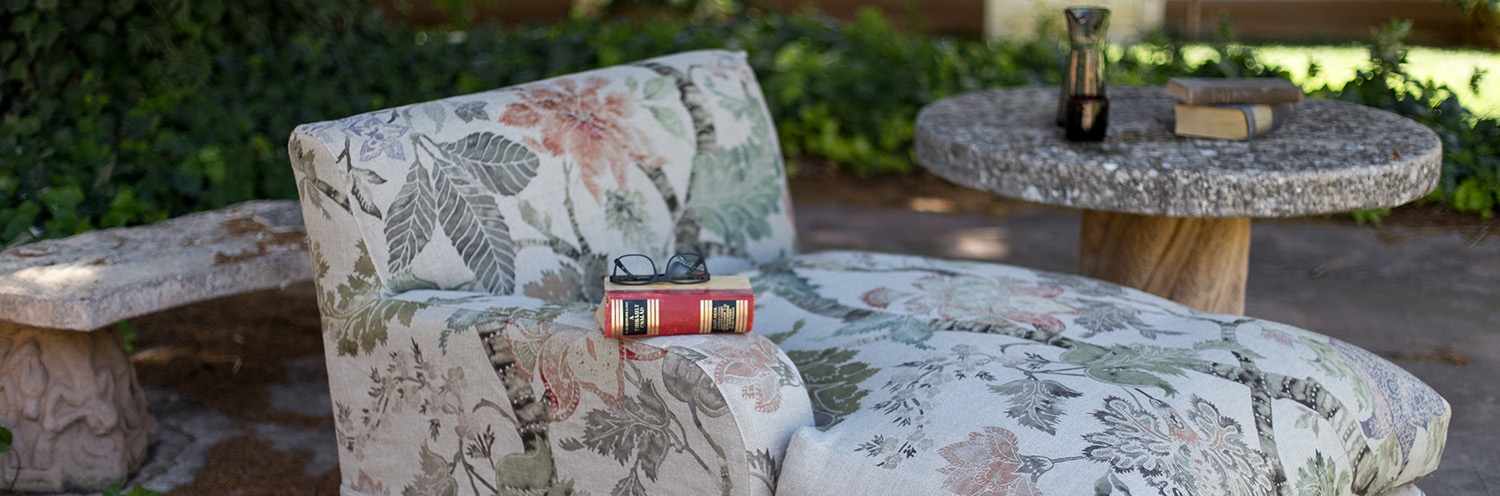# If we were a song, we would be Lennon's IMAGINE, if we were a painting, we would be THE SUNFLOWERS by Van Gogh, if we were a sea, we would be THE MEDITERRANEAN, but, because we are fabrics, we are ZE… conzeta.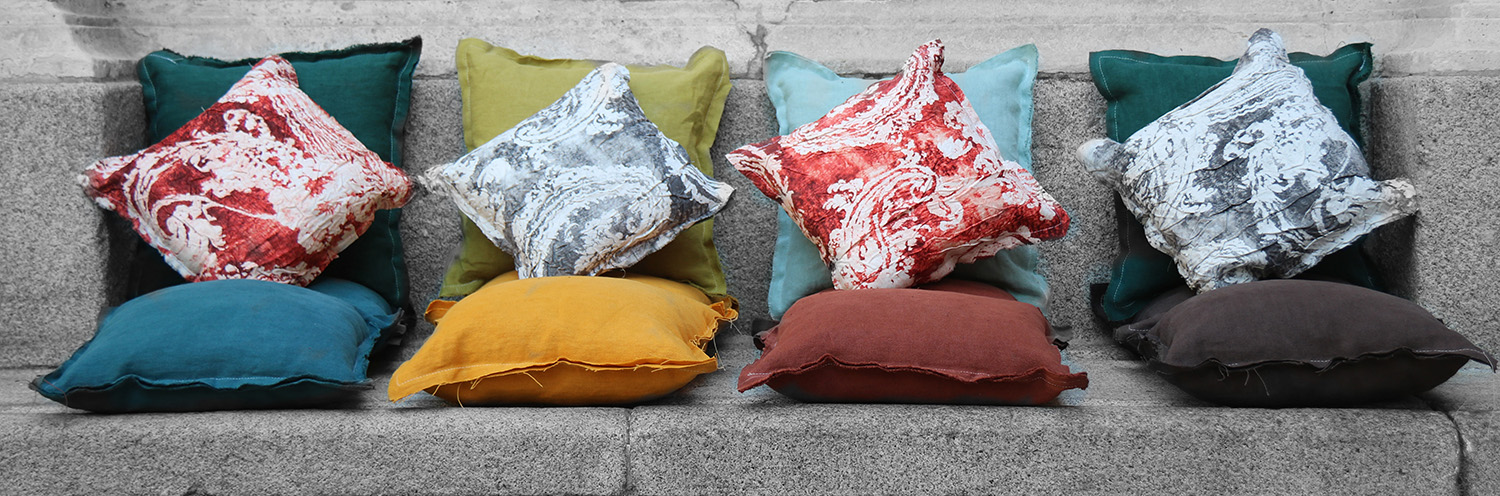# If we were a song, we would be Lennon's IMAGINE, if we were a painting, we would be THE SUNFLOWERS by Van Gogh, if we were a sea, we would be THE MEDITERRANEAN, but, because we are fabrics, we are ZE… conzeta.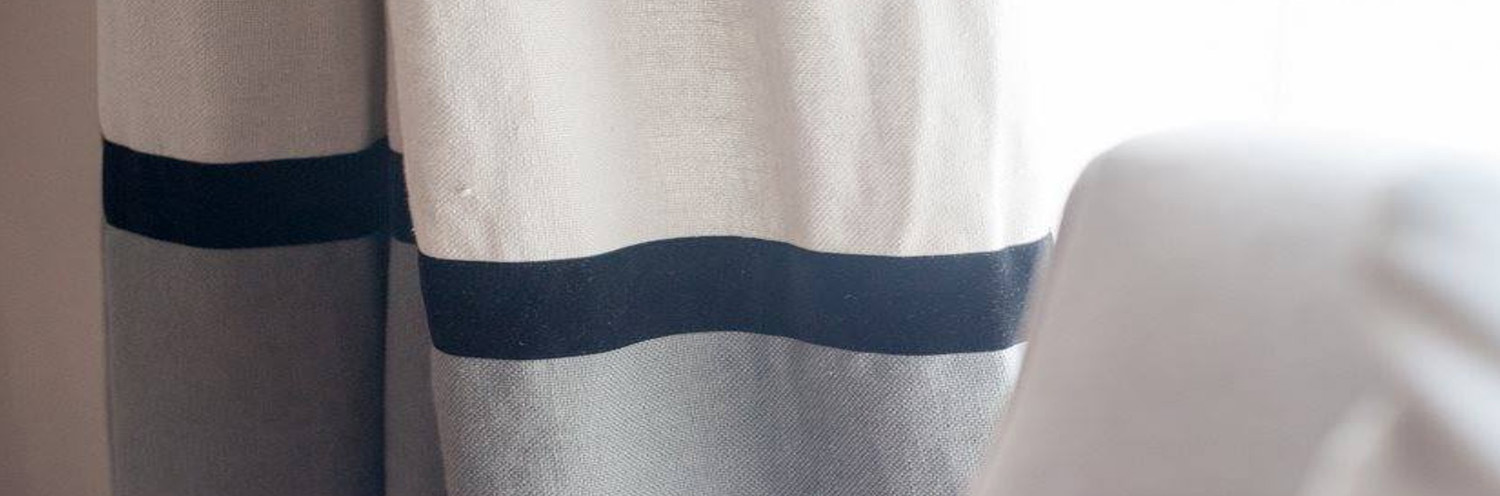# If we were a song, we would be Lennon's IMAGINE, if we were a painting, we would be THE SUNFLOWERS by Van Gogh, if we were a sea, we would be THE MEDITERRANEAN, but, because we are fabrics, we are ZE… conzeta.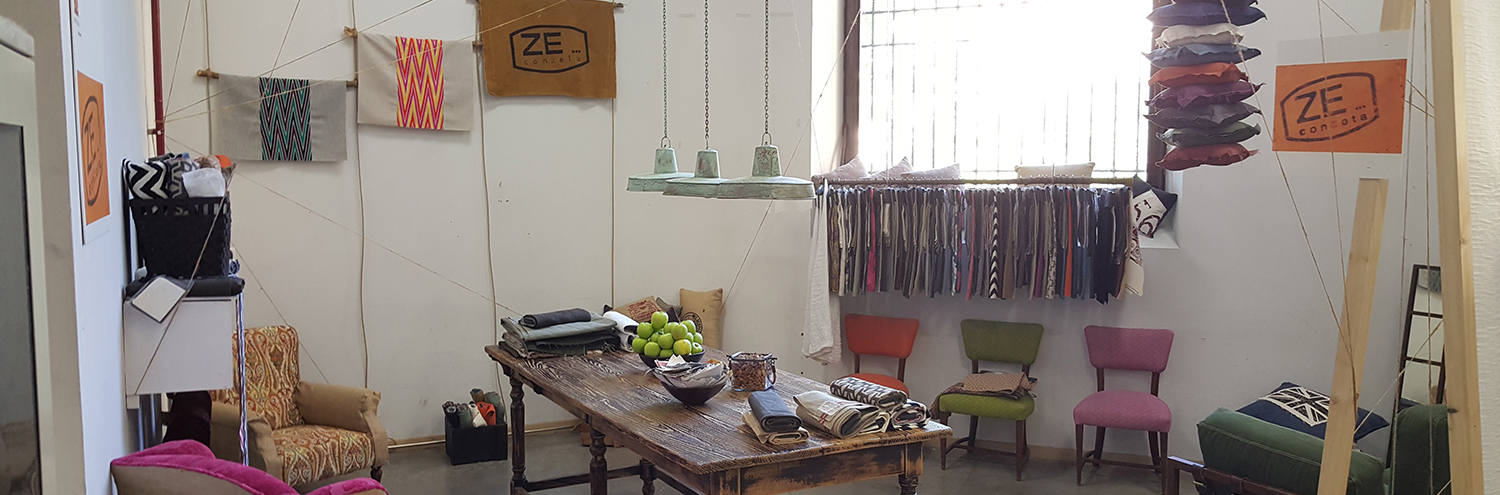# If we were a song, we would be Lennon's IMAGINE, if we were a painting, we would be THE SUNFLOWERS by Van Gogh, if we were a sea, we would be THE MEDITERRANEAN, but, because we are fabrics, we are ZE… conzeta.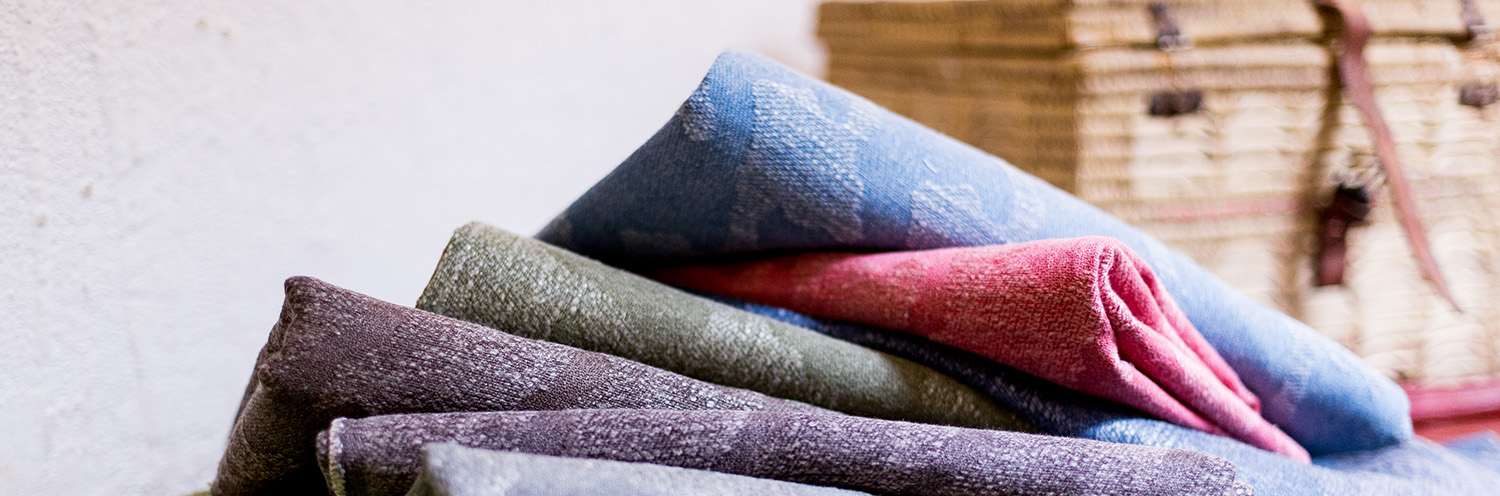# If we were a song, we would be Lennon's IMAGINE, if we were a painting, we would be THE SUNFLOWERS by Van Gogh, if we were a sea, we would be THE MEDITERRANEAN, but, because we are fabrics, we are ZE… conzeta.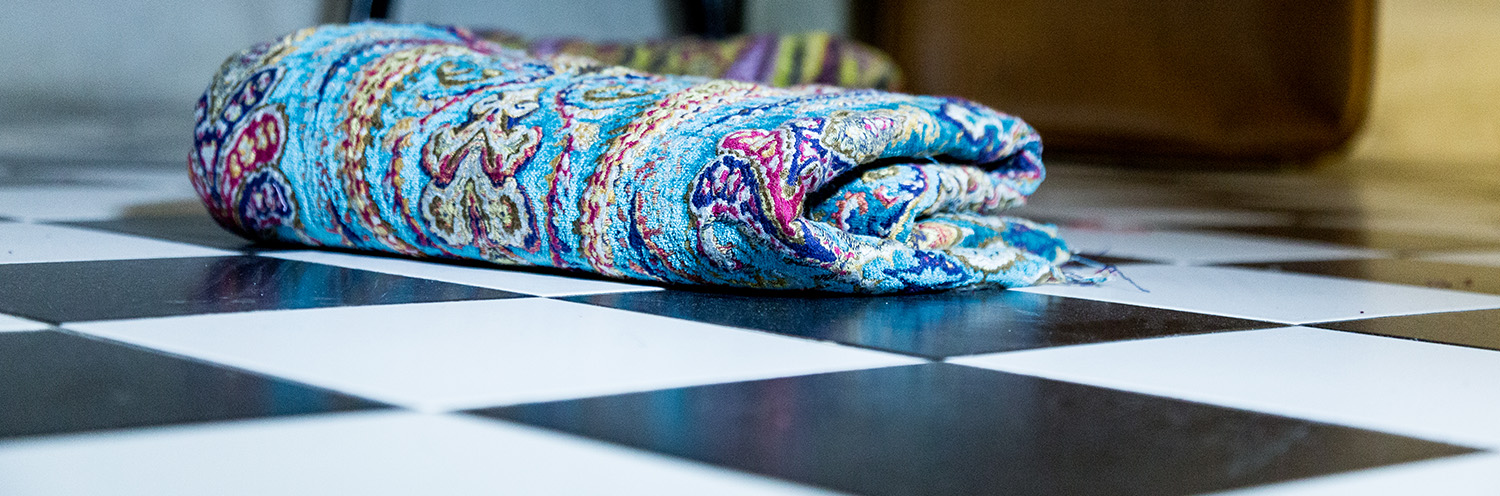# If we were a song, we would be Lennon's IMAGINE, if we were a painting, we would be THE SUNFLOWERS by Van Gogh, if we were a sea, we would be THE MEDITERRANEAN, but, because we are fabrics, we are ZE… conzeta.